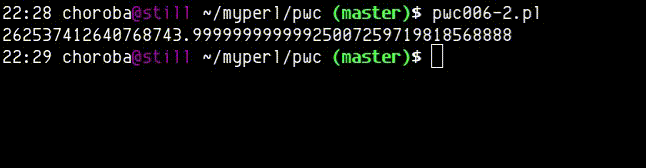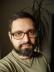## Perl Weekly Challenge 006: Ranges and Ramanujan's Constant

This week, after having read the tasks, I decided to demonstrate the power of CPAN. There already are modules that solve the problems, so the only skill you need is the art of searching CPAN.

## Ranges

Here’s a solution using String::Util::Range. There might be other modules with similar capabilities.

``````#! /usr/bin/perl
use strict;
use warnings;

use Test::More tests => 1;

is compact('1,2,3,4,9,10,14,15,16'), '1-4,9,10,14-16';

use String::Util::Range qw{ convert_sequence_to_range };
sub compact {
my (\$seq_string) = @_;
join ',', @{ convert_sequence_to_range(
array     => [split /,/, \$seq_string],
threshold => 3,
separator => '-')
};
}
``````

## Ramanujan’s Constant

This time, I used Math::BigFloat which is part of the core since 5.0.

``````#! /usr/bin/perl
use warnings;
use strict;
use feature qw{ say };

use Math::BigFloat;

use constant ACCURACY => 64;

my \$r = ('Math::BigFloat'->bpi(ACCURACY)
* 'Math::BigFloat'->new(163)->bsqrt(ACCURACY)
)->bexp;
\$r->precision(-32);
say \$r;
``````
It took me some time to get it right. At the beginning, I used `precision` instead of `accuracy` but I hit the bug #84950: Global precision makes pi into NaN. Also, I wasn’t sure what accuracy is sufficient to get the correct answer. In the end, I verified my solution with
``````my \$r   = 0;
my \$acr = 1;

while (\$r !~ /\..{40}/) {
++\$acr;
\$r = ('Math::BigFloat'->bpi(ACCURACY)
* 'Math::BigFloat'->new(163)->bsqrt(\$acr))->bexp;
print STDERR \$r, " \$acr\r";
}
\$r->precision(-32);
say "\n", \$r;
``````## Leave a comment

### About E. ChorobaI blog about Perl.